# 1st Grade Diagnostic Math Test

Math TestsMany exciting mathematical concepts are introduced to students in the first grade. The ideal math test for 1st grade covers a wide range of topics, including counting, addition, subtraction, measurement, shapes, and size.

Teachers use the first-grade math test to check students’ understanding of their lessons. Most of the time, these tests contain multiple-choice and fill-in-the-blank questions that cover first-grade math concepts.

## The Importance of the Diagnostic Test

When it comes to determining the strengths and weaknesses of students in math, diagnostic tests are essential tools. These tests determine how well first-graders understand important math concepts. The tests can also help teachers determine where students might need more help or instruction.

One of the main advantages of diagnostic tests is that they show how well students master necessary math skills. Thus, teachers can identify the concepts students need to review or learn for the first time by administering a diagnostic test at the beginning of the school year.

Teachers may improve students’ math skills after getting the test results by tailoring instruction and providing specific support. For instance, if a diagnostic test reveals that a student struggles with fundamental addition and subtraction, the instructor can give that student extra attention to assist them in mastering these skills.

Additionally, diagnostic tests can assist educators in identifying gaps in students’ learning that may have emerged over the summer or the previous school year. By administering the test, teachers can identify areas where students may require additional assistance to catch up with their peers.

Diagnostic tests can allow educators to monitor student progress over time. Teachers can observe how students’ math comprehension and skills have improved by administering the same diagnostic test at the start and end of the school year. The teachers can adjust instructions, and additional support can be provided using this information.

The diagnostic math test 1st grade students take helps determine their math strengths and weaknesses and provides instructions to support their success. Teachers can ensure that students get the help they need to do well in first grade math by giving them a diagnostic test at the start of the school year.

A student’s comprehension of fundamental math concepts and skills, such as number recognition, counting, addition and subtraction, measurement, and geometry, is evaluated in 1st grade math test worksheets. Typically, these tests are given to first-graders to assess their progress and pinpoint areas for improvement in their math education.

A primary objective of a first-grade math test is to assess students’ ability to apply fundamental mathematical concepts. Additionally, it assists teachers in determining areas in which students may require additional instruction or support.

Below are first grade math test activities to work on. Before you begin working, print the first grade math test. After that, attempt each question.

### Find a solution to the following:

1. Which number comes after these in the sequence?

14, 15, 16, 17, ____

1. 18
2. 20
3. 19
4. 12
2. How many cents are there in a dime?
1. 15 cents
2. 10 cents
3. 12 cents
4. 25 cents
3. Take a look at the clock below. What time is it right now?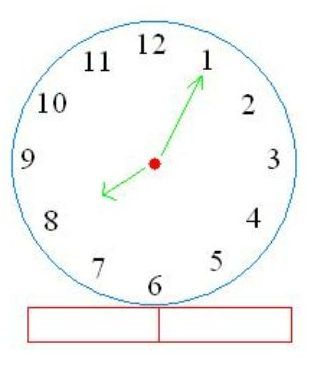4. Luke had twelve pencils, but he gave five to his closest friends. How many pencils does Luke currently have?
5. Please fill in the two omitted numbers.

34, 36,____, 40, ____

6. What is the amount of cents in a quarter? How many nickels are there?
1. 20 cents and 5 nickels
2. 25 cents and 4 nickels
3. 25 cents and 5 nickels
4. 20 cents and 4 nickels
7. John ate five pizza slices and gave his friend Anna four. How many pieces did the pizza come with?
8. Take a look at the image below. Which rectangle sits above number 40?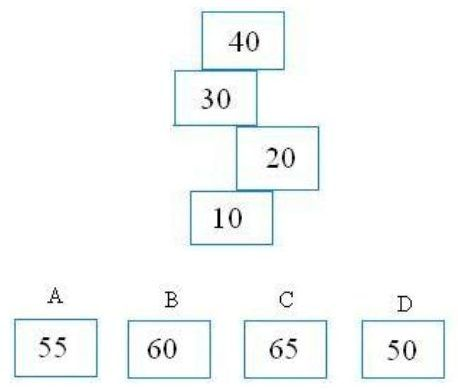9. Perform the subtraction that follows:10. Determine whether the numbers on the left-hand side are lower or higher than the ones on the right. You should replace the question mark (?) with the greater than or less than symbol.

4       ?      2

5       ?      7

11. a) Color one-quarter or one-fourth of the shapes on the right and half of the shapes on the left:

b) Color the triangle red, the rectangles yellow, the circle orange, and the other figures any color you like.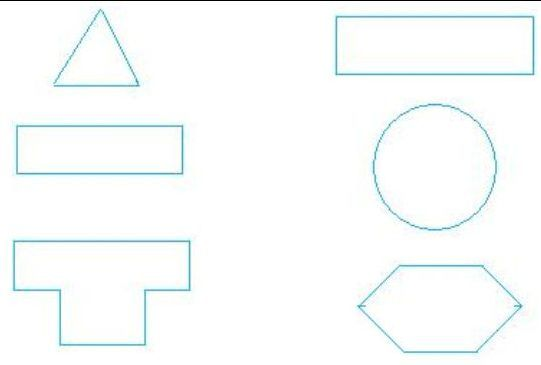12. Which digit is in the tens place for the number 56? What is their location?
13. Make the additions and subtractions listed below.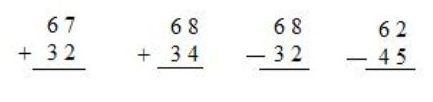14. What time is shown below?15. In words, write the following numbers: 2 _____________, 7 _____________, 14 _____________, and 24 _____________.
16. a) What is the name of a geometric figure that looks like a ball? A can of soda? _______________

b) Which well-known figure can be found in Egypt? Something you serve with your ice cream? _________________

17. Please fill in the blanks for the missing number 65, 60, ____, 50, 45, and ____________
18. What is the length of the line in red below?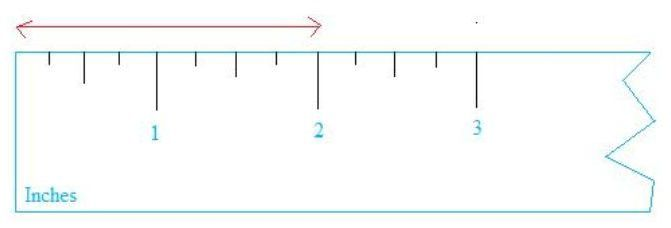19. In fifty cents, how many dimes are there?
20. Math Challenges: Who am I?

5 + 5 is less than I am. 5 + 8 is greater than I am.

I am an odd number; if you subtract 10 from me, you get a number greater than 1.

## Printable 1st Grade Math Tests

Numerous educational math websites and publishers provide the ideal 1st grade math assessment test in printable format for students in the first grade. They may incorporate good tips, like articles about estimating or tackling word problems. Multiple-choice, matching, and fill-in-the-blank questions are examples of questions included in first-grade math assessment tests.

## What Math Skills Are Tested in the 1st Grade?

Students learn a lot of new essential math concepts in the first grade, which makes this period an exciting time for kids. 1st grade math test free resources cover various topics and skills, including counting, essential addition and subtraction, geometry, and measurement.

Number sense is one of the crucial math skills tested in the first grade. It requires students to be able to count, compare, and arrange numbers as well as comprehend and use numbers in various settings. Students may, for instance, be required to calculate a set of objects or determine which number comes first or last.

Essential addition and subtraction are other necessary math skills tested in the first grade. The ability to solve addition and subtraction problems, such as 1 + 2 = 3 or 4 – 2 = 2, are expected of students. They should also be able to solve these problems with manipulatives like blocks or counters.

Students in the first grade are expected to have a fundamental understanding of geometry and measurement in addition to basic math skills. It includes identifying and describing common shapes like squares, triangles, and circles as well as measuring length, height, and weight in basic units like inches and centimeters.

## How to Prepare for the 1st Grade Math Test?

Taking a math test can be nerve-wracking for some students, but parents and teachers can help them feel more confident and prepared for their first grade math test by taking a few simple steps.

First, go over the math skills and concepts that are tested. You can utilize cheat sheets, games, or other intelligent apparatuses to help understudies practice and survey the material. Review the resources with your child or students before the test to help them become more familiar with them.

Then, encourage your child or student to practice solving math problems similar to those given at the exam. This way, they can learn the skills and methods they need to solve problems quickly and accurately. You can use materials like math workbooks, online materials, and other resources as additional practice opportunities.

In addition, create a welcoming and encouraging learning environment. Students can feel more confident and less anxious about taking a math test if the classroom is calm and supportive. To help students correctly solve problems, encourage them to take their time and use strategies like rereading questions or checking their work.

Lastly, make it clear to students that making mistakes is a normal part of learning and that they can improve their understanding of math concepts by learning from mistakes. Please encourage them to see difficulties and failures as opportunities for development and education.

## Look Online for More Math Help

If your child is having trouble with this diagnostic testing, make sure to apply for the Brighterly’s evaluation lesson. Brighterly’s lessons are based on generally-accepted 1st grade math test online standards that guarantee positive learning outcomes. This user-friendly interactive educational platform with engaging mechanics appeals to first grade students and prepares them to ace any 1st grade math test.

Children score higher on the knowledge evaluation test administered by Brighterly than on the placement test. These results show that kids learn in a fun way when they play games, learn from experienced teachers, and learn online one-on-one.

Book 1 to 1 Demo Lesson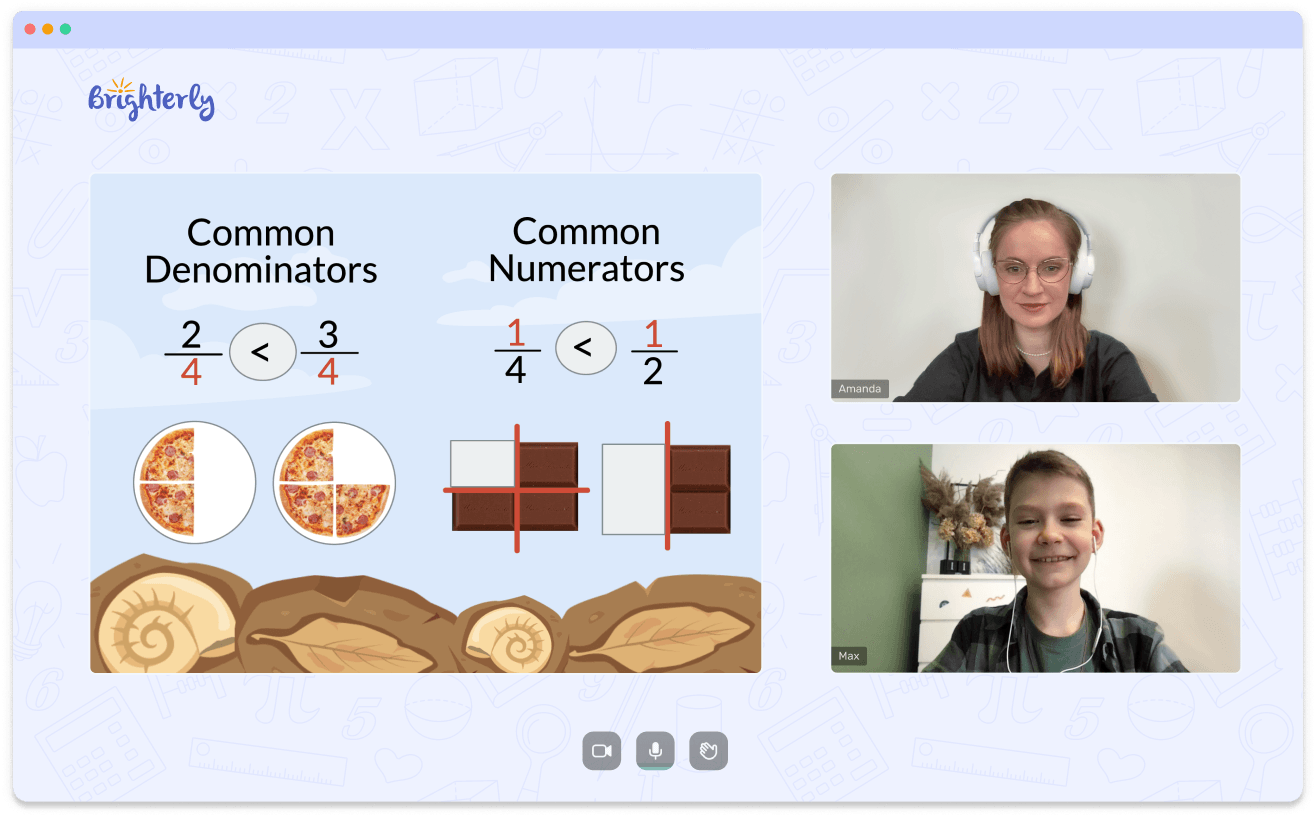• We help children understand Math concepts instead of memorizing.
• We will adapt to your child needs to mprove math performance.

Is Your Child Struggling With Math?
Book 1 to 1 Demo Lesson